# MAIN

• Introduction

• Materials and Methods

•   Sample materials and treatment

•   Measurements of acid value

•   Measurement of near-infrared transmission spectrum

•   PLSR modeling

•   Optimal variable selection

• Results and Discussion

•   Titration analysis of soybean oil

•   Near-infrared transmission spectra of soybean oil

•   Estimation of PLSR models for preprocessing methods

•   Comparison of PLSR models for variable selection methods

• Conclusions

• Conflict of Interest

## Introduction

It is well established that edible oils have a critical effect on the taste and mouthfeel of foods, whilst enhancing the nutritive value of the food. Edible oils, as one among the three major nutrients, is a high-energy source that contributes to the human body by composing the cell membranes as the fat-soluble carriers and protecting the hypodermic tissues and organs (May et al., 1983; Lee et al., 1997); however, being used in most homes, restaurant business, and food processing industry, it undergoes changes in smell and taste owing to the chemical or microbial effects during its storage and processing. These changes reduce the nutritive components of the edible oils, lower the quality of the processed foods, and produce toxic agents in humans occasionally. This phenomenon of deterioration of edible oils is known as rancidity (Chung and Choe, 2003; Summerfield and Tappel, 1984).

Especially, an oxidation process, which is a representative cause of rancidity, can destroy the essential fatty acids and produce toxic agents and oxidized polymers; therefore, oxidation of edible oils is considered to be critical in terms of palatability, nutritional quality, and toxicity (Choe and Min, 2006). In particular, soybean oil, which is commonly used for frying, exhibits a low oxidative resistance when heated for a long time or repetitively because it contains a lot of unsaturated fatty acids (Park et al., 2003).

Recently, there have been several studies on spectroscopy techniques to estimate the properties of agricultural products or foods (Ben-Dor et al., 1999; Choe et al., 2008). Particularly, a study based on near-infrared spectroscopy (NIRS) has been widely recognized because the NIR region, by virtue of its wavelength (780-2,500 nm), possesses specific information of the test object (ASTM international, 2000). It is known that each functional group (O-H, C-H, and N-H) in a molecule has characteristic absorption frequency bands in the NIR spectrum owing to its vibrational characteristics. Therefore, the exact wavelength at which a vibration happens can be observed by the strengths of the bonds included. Moreover, a near-infrared spectroscopy technique has some advantages in terms of its relative high energy signal compared to far-infrared signal as well as its simple configuration of detector and light source which induce its easy implementation. In addition, the composition of NIRs such as fiber-optic cable, light source, and detector are known to be easy to operate compared to mid-infrared or far-infrared spectroscopic systems.

Despite the advantages described above, the NIR spectra may contain complex and overlapped frequency bands that, accordingly, necessitate additional signal processing computations to analyze the measured spectra. Since the 1990s, a multivariate analysis based on Chemometric method was used in the analysis of spectral features which related to the properties of object (Kim et al., 2008; Kawano et al., 1992). The most representative methods are multiple linear regression (MLR), principal component regression (PCR), and partial least-squares regression (PLSR) (Dull et al., 1992). Among these chemometric methods, PLSR is known to be more robust to reach minimum error with fewer variables than MLR and PCR (Geladi and Kowalski, 1986; Helland, 1988). However, as the spectra measurements are prone to noise from instruments and their environment, suitable preprocessing methods are being developed for removal of noise (Porep et al., 2015; Rinnan et al., 2009). Herein, we also discuss the optimal variable selection methods that are critical to build a stable and practical model because the developed model includes core subsets of optimal spectra frequencies for better prediction (Sarathjith et al., 2016; Zhang et al., 2017). Previous studies showed that the removal of redundant and noisy wavelengths can improve the prediction performance of a model and reduce its complexity (Kamruzzaman et al., 2013; Kandpal et al., 2016); therefore, we employed four kinds of variable selection method and demonstrated the performance enhancement of the developed prediction models (Lee et al., 1998; Mehmood et al., 2012).

The objective of this study is to develop a non-destructive rancidity evaluation technique of a soybean oil using the near-infrared spectroscopy techniques by using partial least squares regression and optimal wavelength selection methods. Soybean oil samples were deteriorated artificially by boiling them, and titrimetric analysis was used to obtain the reference acid value of each sample. PLSR multivariate analysis with several preprocessing techniques has been employed to quantitatively predict the acid value of soybean oil. In addition, four kinds of optimal variable selection method were used to reveal the optimal wavelength range through model development, and the model performance was also evaluated in conformity with the variable selection methods.

## Materials and Methods

Sample materials and treatment

Soybean oils (CJ CheilJedang Corp., Korea) were obtained from a local market and were boiled using an oil bath (WHB-11, DAIHAN Scientific, Korea) to prepare artificially deteriorated test samples. Twelve soybean oil samples, which were kept in tempered glass bottles, were heated at 180°C for 14 days for 4 h every day using the oil bath, and a sample of soybean oil (10 g) was collected ten times every day for titrimetric analysis test and transmission spectra measurements.

Measurements of acid value

The acid value (AV) of the soybean oil samples was determined using the method of the American Oil Chemists’ Society (AOCS, 1977) with suitable modifications. 1 g of the sample was placed in a flask and dissolved in 20 ml of an ether:ethanol solution (2: 1, v/v) while shaking. 1% of phenolphthalein solution was added as an indicator, and titrated with 0.1 N KOH solution until the red color was maintained for 30 s. The formula for calculating the acid value is shown in equation (1).

$AV=\frac{5.611×A×F}{W}$    (1)

where AV is the acid value, A denotes the volume of KOH (mL), 5.611 is a constant value with equivalence of mass of 0.1 N KOH, W is the weight of sample, and F is the normality of the standard KOH solution.

Measurement of near-infrared transmission spectrum

The soybean oil samples were placed in a plastic cuvette, and the near infrared spectra were measured in a wavelength band of 900-1,700 nm using a near infrared spectrometer (CDI-NIR128, Control Development Inc., USA). The light source was a tungsten halogen light source (LS-1, Ocean optics, USA). A schematic diagram of the measurement setup is shown in Figure 1.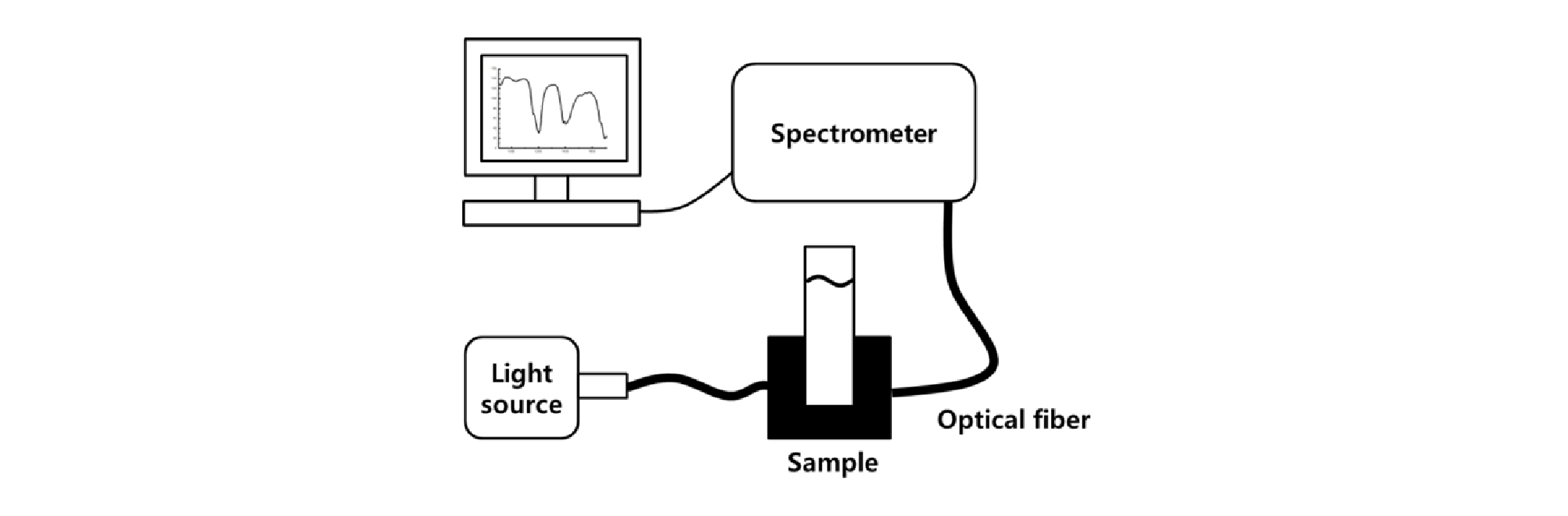##### Figure 1.

Schematic of near-infrared transmission spectrum measurement.

A dataset comprising 140 sets of readings of NIR spectra was obtained by acquiring 10 samples every day for 14 days, whereby daily sampling and measurement were performed in triplicate. The measurement exposure time of the instrument was set to 50 ms, and the measured spectrum was calibrated using equation (2) to convert the measured signal to transmittance. The white reference signal was measured on a plastic cuvette containing no sample, and the dark reference signal was measured in a dark room without light.

$transmittance\left(%\right)=\frac{{S}_{i}-D}{R-D}×100,$    (2)

where Si is the raw transmittance of the samples, and D and R represent the raw transmittance of the dark and white referenced spectra, respectively.

PLSR modeling

Spectral preprocessing methods were used to correct the error factors such as noise and light scattering during the measurement of spectra. In this study, two types of scatter correction methods such as multiplicative scatter correction (MSC) and standard normal variate (SNV), three kinds of normalization methods including maximum, range and mean normalization, and Savitzky- Golay 1st and 2nd order derivative methods were employed and evaluated. The prediction model for the acid value of the soybean oil samples was constructed using PLSR which is defined in equation (3). Algorithms for preprocessing and regression modeling were developed using commercial software (Matlab ver. R2016, MathWorks, Natick, MA, USA).

(3)

where X is the n by m matrix of predictors, Y is the n by p matrix of responses, T is the n by 1 matrix of the score matrix, E and F are Gaussian error terms, and P and Q are the m by 1 and p by 1 loading matrices, respectively.

The selection process of latent variables number, a new variable in the PLSR model, is an important process in PLSR modeling. In this study, we randomly sampled the data 100 times from a cross-validation set of 100 samples to 7:3 (calibration set:validation set) and selected the optimal number of latent variables through cross validation. The model was constructed from the cross-validation sets by applying the optimal number of latent variables, and the accuracy of the model was evaluated using the test set of the remaining 40 samples. The performance of the constructed model was evaluated by root mean square error (RMSE) calculated as described in equation (4).

$RMSE=\sqrt{\frac{\sum _{i=1}^{m}{\left({y}_{obs}-{y}_{pre}\right)}^{2}}{m}}$    (4)

where RMSE represents the sample standard deviation of the differences between predicted values and observed values. yobs and ypre are the observed and predicted values, respectively. m is the number of data.

Optimal variable selection

PLS-Beta-Bootstrap

Bootstrap was invented to obtain new datasets through random resampling of a sample population and establishing confidence intervals by taking statistical values from the sampled datasets (Bickel and Freedman, 1981). The PLS-bootstrap method resamples multiple times for a measured spectral dataset and constructs each PLSR model for the resampled datasets. From the distribution of the regression coefficient (beta coefficient) for each PLSR model, the confidence interval of the beta coefficient of each variable is calculated, and if there is a 0 in the confidence interval, the corresponding variable is removed. The confidence interval in this process is calculated as equation (5) (Lazraq et al., 2003).

${I}_{k}={\overline{)b}}_{k}±c{s}_{k},$    (5)

where Ik represents the confidence interval, ${\overline{)b}}_{k}$ is the mean of the beta coefficients at the kth variable, c is a constant that determines the confidence interval, and Sk is the standard deviation of the beta coefficients at the kth variable.

In equation (5), the constant c is explicitly determined regarding the level of significance. A higher significance level could select fewer wavelength bands. In this study, the number of resampling is set to 1,000, and the model accuracy with the selected wavelength is evaluated by changing the c values (Lazaraq et al., 2003). In our study, the wavelength was selected by setting the number of resampling times to 1000 and c to 1.

PLS-VIP (Variable Importance in Projection)

Variable importance in projection (VIP) method is a technique that considers the contribution of each variable reflected in weight, which is a PLSR parameter. The VIP value is calculated as written in equation (6).

(6)

where vj represents the VIP value, p is the number of variables, SSa is the sum of squares explained by the ath component, wa is the weight of the ath component, and wak is the weight of the ath component at the kth variable.

Hence, the vj is a measure of the contribution of each variable according to the variance explained by each PLS component where (wakwaǁ2) represents the importance of the kth variable. The wavelength selection using the VIP method is performed by setting a threshold value and selecting a variable having a VIP value equal to or higher than a threshold value. In general, a threshold value of 1 is used (Mehmood et al., 2012). In this study, the model was constructed by setting the threshold value to 1.

## Results and Discussion

Titration analysis of soybean oil

The rancidity extents of the artificially deteriorated soybean oil samples were estimated as the acid values through titration test, and the results are summarized in Figure 2. The soybean oil samples were boiled at 180°C for 4 h every day during fourteen days and the relative humidity (RH) of the laboratory was controlled at 20% RH. For statistical analysis of the acid values, all the treatment groups were compared using one-way analysis of variance, with a post hoc Student-Newman- Keuls (SNK) multiple comparison test. Analysis of variance with a post hoc SNK test for multiple comparisons was performed using the commercial statistics software SPSS (ver. 18, IBM, Armonk, NY, USA) to verify the significance of differences between the acid values of each treatment group. The differences between means were assumed to be significant if the p value was less than 0.05. As shown in Figure 2, there were significant different rises in the AVs from six days during whole test periods. The result of a post hoc SNK multiple comparison is shown in Figure 2, and averaged acid value was considered to be significantly different if the SNK test yielded a p value of less than 0.05. Different letters in each column bar of Figure 2 indicate significant differences (p<0.05). The acid value of soybean oil samples which artificially treated for six days was 0.6408 ± 0.0787 mg/g, and the acid value of soybean oil samples treated for whole test period (14 days) was 6.2147 ± 0.1804 mg/g.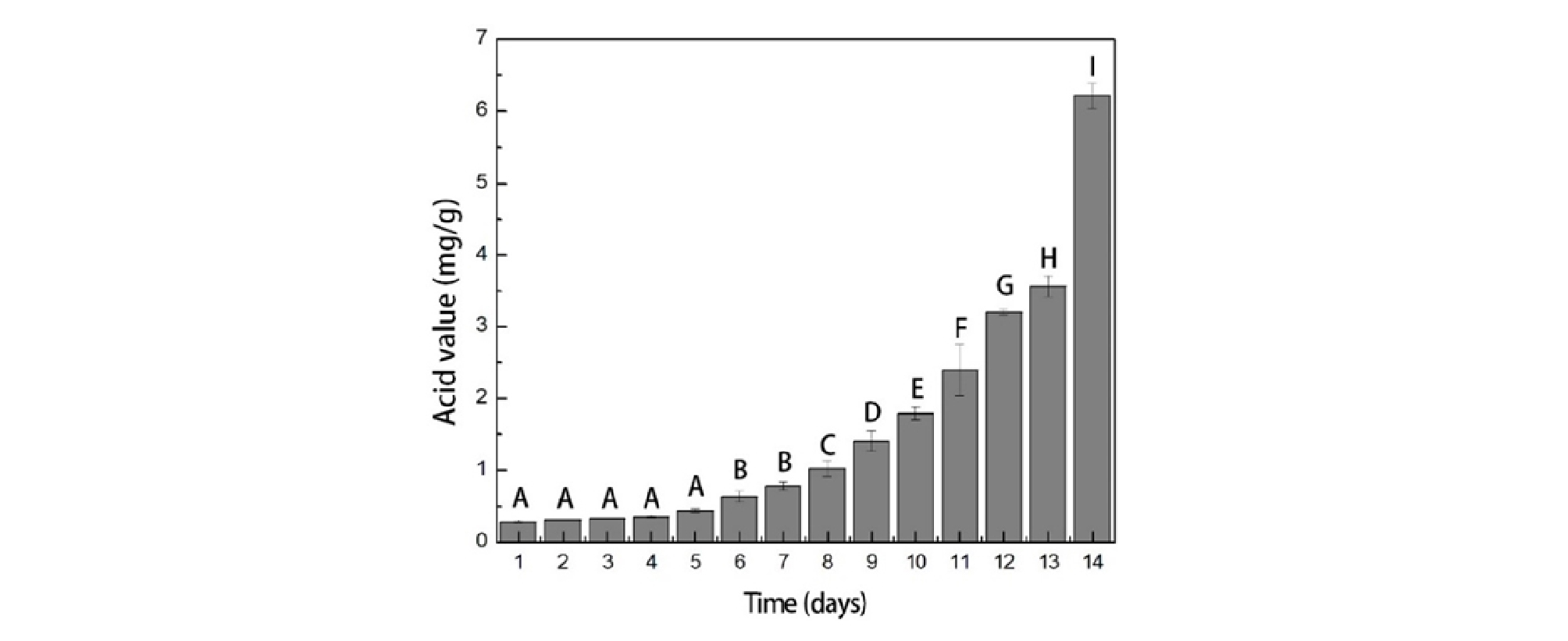##### Figure 2.

Acid values of soybean oil samples for time periods.

Near-infrared transmission spectra of soybean oil

Figure 3 shows the transmission spectra of soybean oil samples before preprocessing applied. As shown in Figure 3, the transmission spectra decrease with increasing test periods as well as high absorptions at around the wavelengths of 1,200 and 1,400 nm. A degradation in a vegetable oil can cause the increase of –ROOH, −OH, =O, and –CHO groups by the formation of hydroperoxide and carbonyl compounds, and also the NIR spectra are absorbed at 1,195 nm and 1,410 nm by the second and first overtone of the C-H and O-H stretch vibrational transitions, respectively; therefore, the sharp decrease of transmission spectra at and around 1,200 nm and 1,400 nm might be demonstrated on the basis of previous study described above.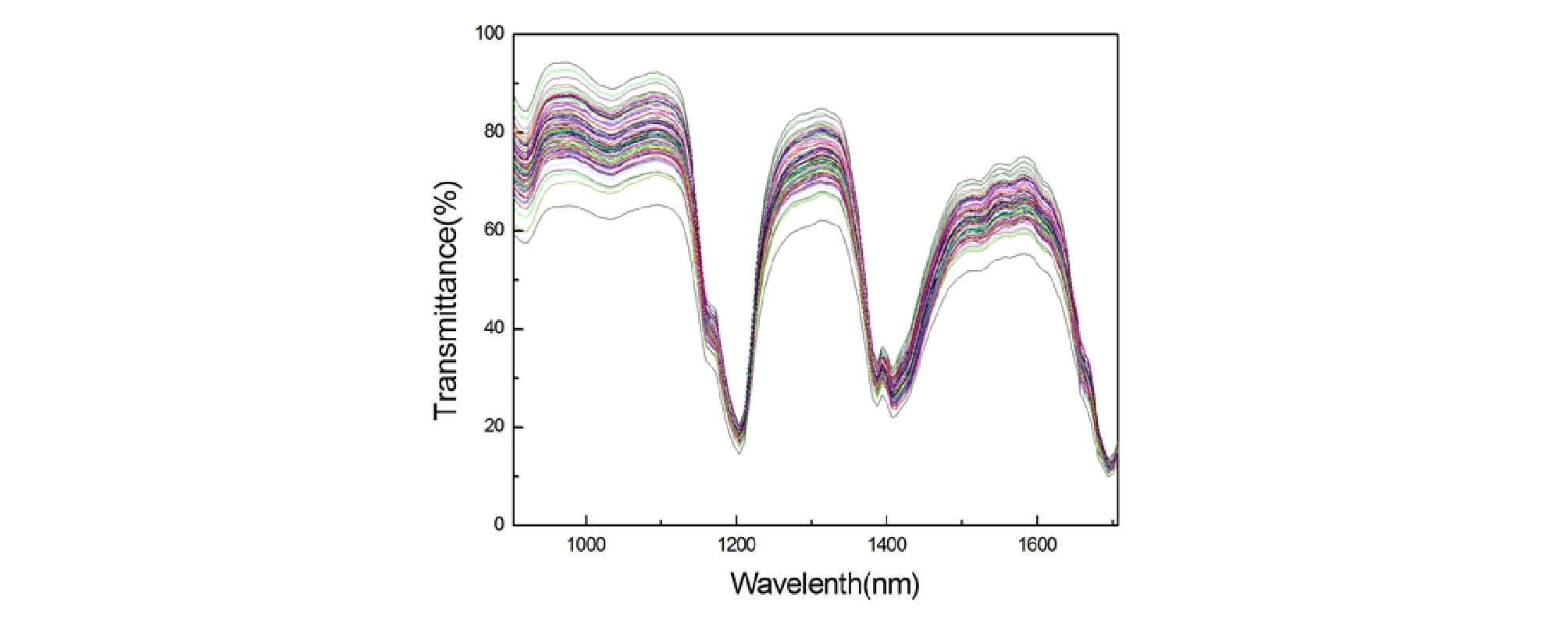##### Figure 3.

Near-infrared transmission spectra of soybean oil.

Estimation of PLSR models for preprocessing methods

Seven kinds of preprocessing methods were employed to enhance the performance of the prediction model, and Table 1 shows the results of the PLSR models for each method. The performance of the developed model was evaluated by the coefficient of determination (R2) and RMSE, between the titration test results and the prediction values. The test results of the regression models showed that Savitzky-Golay 2nd order derivative method showed the highest performance (Rt2: 0.8711, RMSEt: 0.6680), and followed by the regression model with mean normalization preprocessing (Rt2: 0.8650, RMSEt: 0.6835). Figure 4 shows the prediction result of two most highly correlated PLSR models for AV estimation.

Table 1. Performance comparison of PLSR models for different preprocessing methods

 Preprocessing methods LV Cross-validation Test R2 RMSE R2 RMSE raw data 9 0.8807 0.5005 0.8650 0.6837 MSC 9 0.8826 0.4965 0.8385 0.7477 SNV 9 0.8872 0.4868 0.8366 0.7521 Savitzky-Golay 1st order derivative 7 0.8619 0.5384 0.8114 0.8079 Savitzky-Golay 2nd order derivative 7 0.9088 0.4375 0.8711 0.6680 normalization maximum 10 0.8864 0.4885 0.8510 0.7182 mean 9 0.8801 0.5018 0.8650 0.6835 range 9 0.8823 0.4973 0.8416 0.7406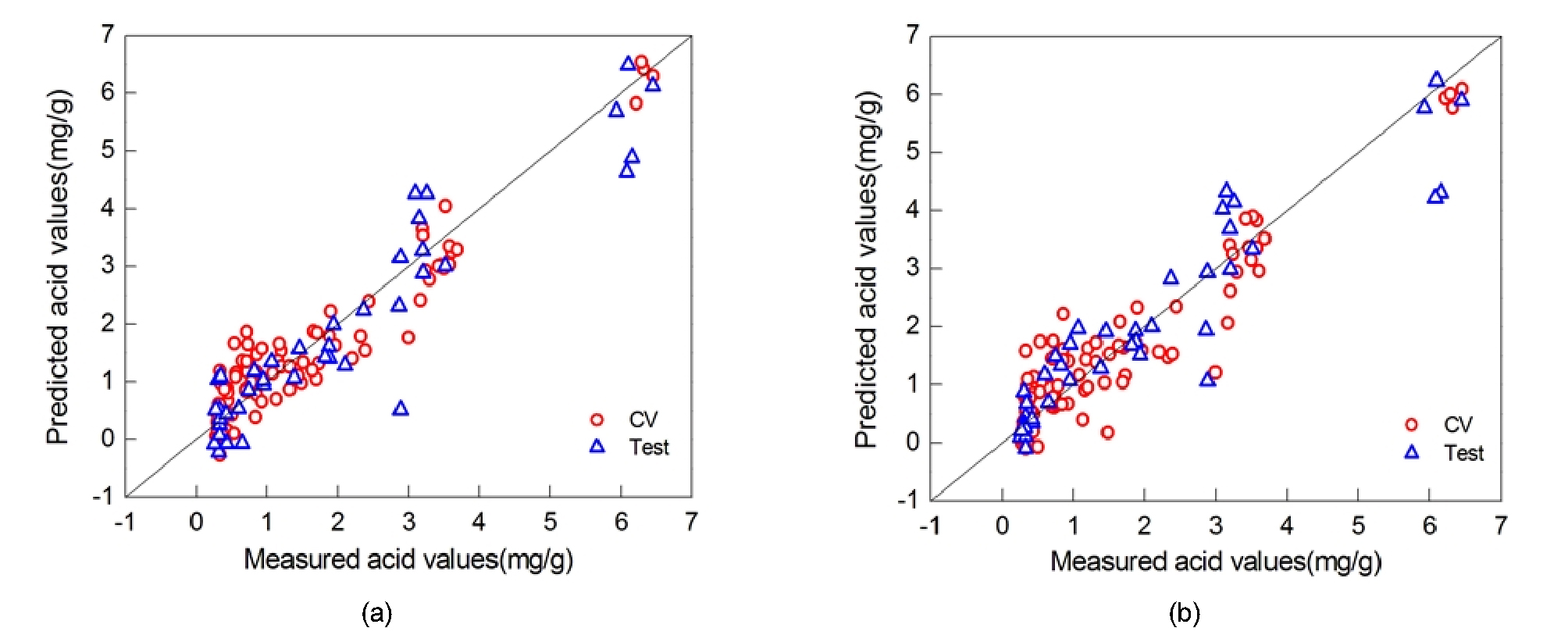##### Figure 4.

PLSR results for (a) SG 2nd, and (b) mean normalization preprocessing methods.

Comparison of PLSR models for variable selection methods

In this study, two kinds of variable selection methods were employed to select the optimal wavelength bands from whole wavelength dataset (900-1,700 nm). NIR dataset which treated by Savitzky-Golay 2nd order derivative method was used in the works of optimal variable selection because the PLSR model which developed by the preprocessing method of Savitzky- Golay 2nd order derivative method showed the highest performance in the acid value of soybean oil samples. Then, the selected spectra data set was used as a variable of the PLSR model. Figure 5 shows the first and second weight vectors with respect to equivalent latent variables of the PLSR model which obtained from the entire wavelength’s spectra. The weight vector can imply the correlation between the measured spectrum and calculated latent variables through PLSR analysis. It revealed how much the measured spectrum contributed to the formation of corresponding latent variables. As shown in Figures 5 (a) and (b), the highest peaks are observed around the wavelengths of 1,150 nm and 1,450 nm, which are highly correlated with the largest absorption by the second and first overtone of the C-H and O-H stretch vibrational transition, respectively, caused by the deterioration of soybean oil.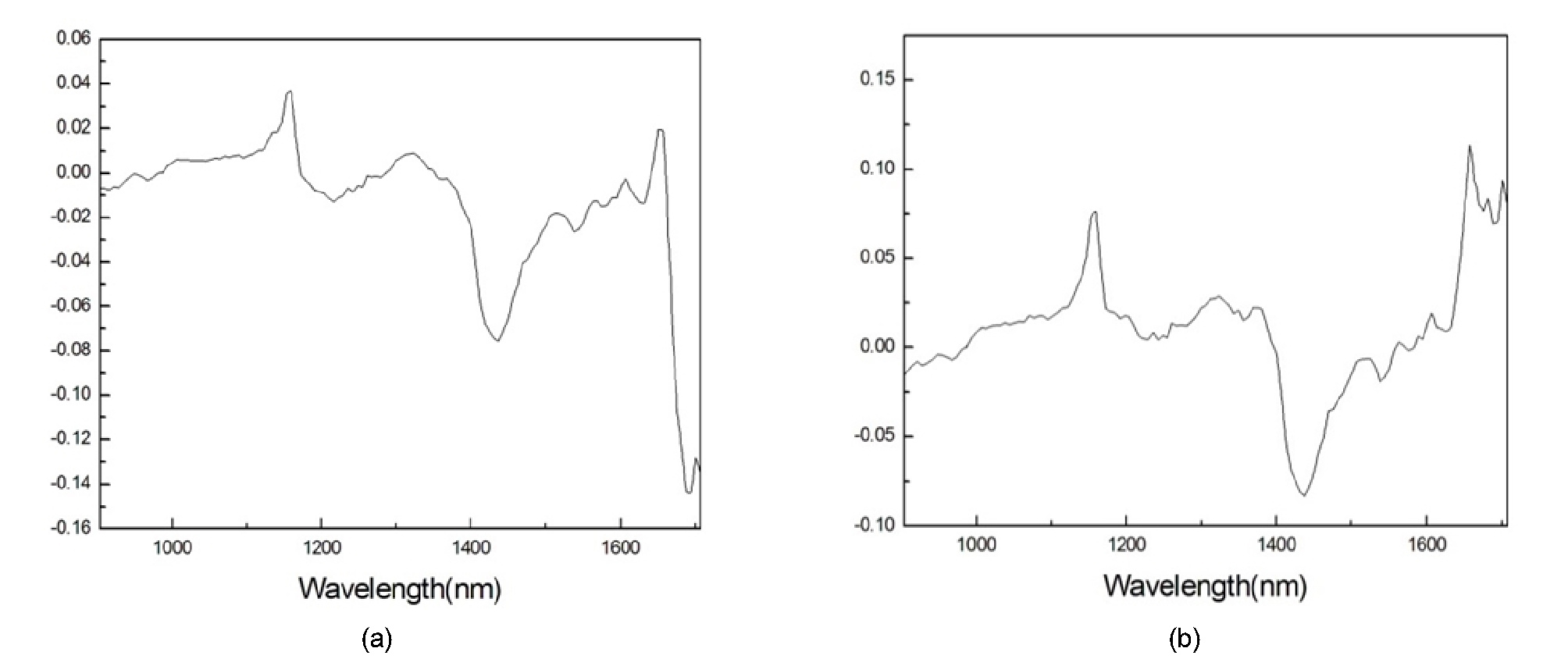##### Figure 5.

Two weight vectors with respect to equivalent latent variables of the PLSR model: (a) First weight vector and (b) Second weight vector.

Figure 6 shows the optimal wavelength bands determined by two different variable selection methods. By setting the significant level constant c as 1, the extracted wavelength bands were relatively evenly distributed over the entire range, as shown in Figure 6(a). VIP method was implemented with threshold values v set to 1.0, and the extracted wavelength bands were relatively evenly distributed over the entire range, as shown in Figure 6(b).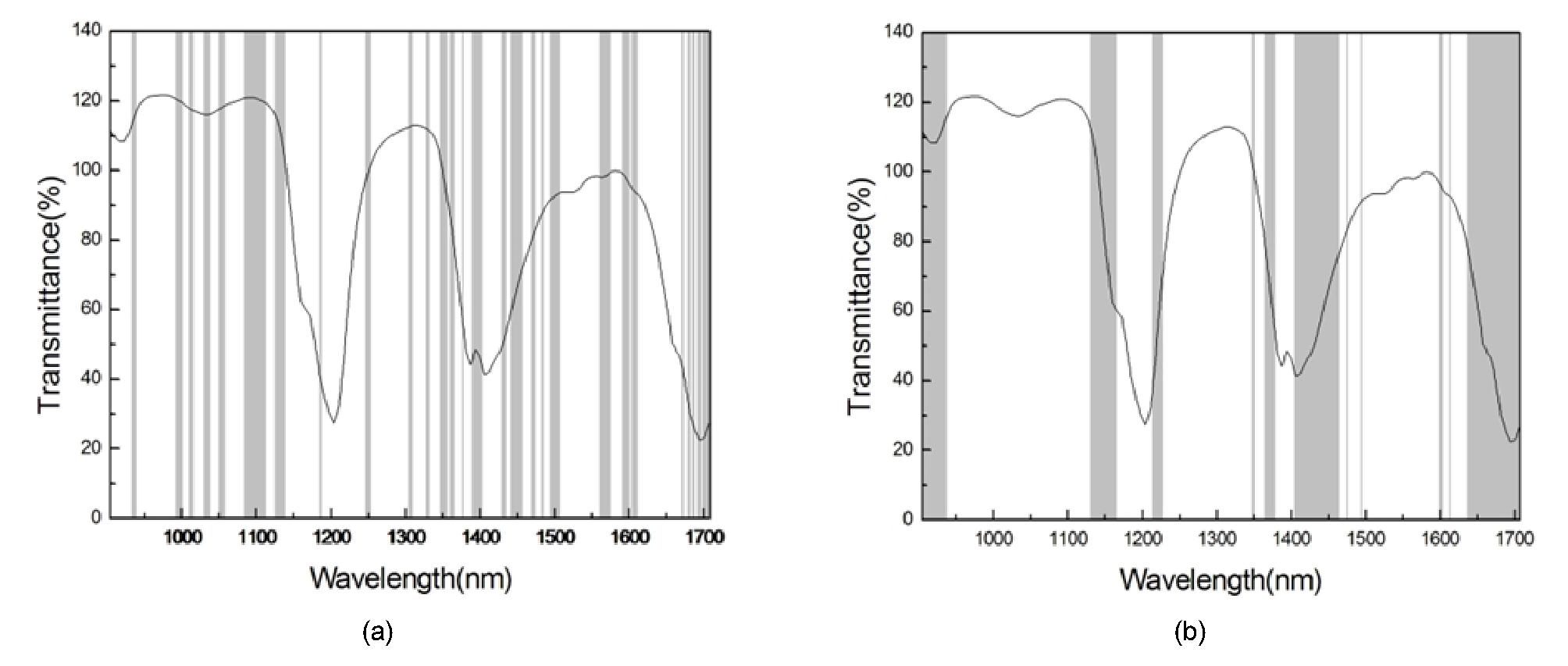##### Figure 6.

Selected wavelengths by (a) bootstrap (c = 1.0, N = 210) and (b) VIP (v = 1.0, N = 234) methods for transmittance spectrum.

Table 2 shows the cross validation and test results of the PLSR models developed with the optimal variables. Prediction performance of each model was compared, and the PLSR model with whole spectra of wavelength is also described. When the bootstrap was used, the PLSR model with spectra data (c = 1.0) corresponding to 210 numbers of wavelengths showed good performance (Rt2: 0.8403, RMSEt: 0.7436) when compared to the full PLSR model (Rt2: 0.8711, RMSEt: 0.6680). The VIP variable selection method presented that the prediction performance of the PLSR model with spectra dataset which corresponding to 234 numbers of wavelengths is similar (Rt2: 0.8081, RMSEt: 0.8150) to that of the full PLSR model. Thus, the prediction performance of the PLSR models with reduced spectra owing to the variable selection methods showed similar performance with the full PLSR model, but not an enhanced performance; however, these optimal variable selection methods can be successfully used to enhance the efficiency of the model runtime and the data saving effectiveness of the measuring device, while providing a good prediction accuracy by way of selecting the best spectra optimally. Figure 7 shows the prediction performance of the PLSR models that were developed using the optimally selected spectra. As shown in Figure 7, whole samples were fairly well predicted compared to the result of full PLSR model shown in Figure 4. Furthermore, the results confirm the reliability of the model to predict the acid value of soybean oil.

Table 2. Comparison of the PLSR model results for different optimal wavelength selection methods

 Wavelength selection method No. of variables LV Cross-validation Test R2 RMSE R2 RMSE Full PLSR 804 9 0.9088 0.4375 0.8711 0.6680 Bootstrap 210 8 0.8657 0.5311 0.8403 0.7436 VIP 234 8 0.8672 0.5281 0.8081 0.8150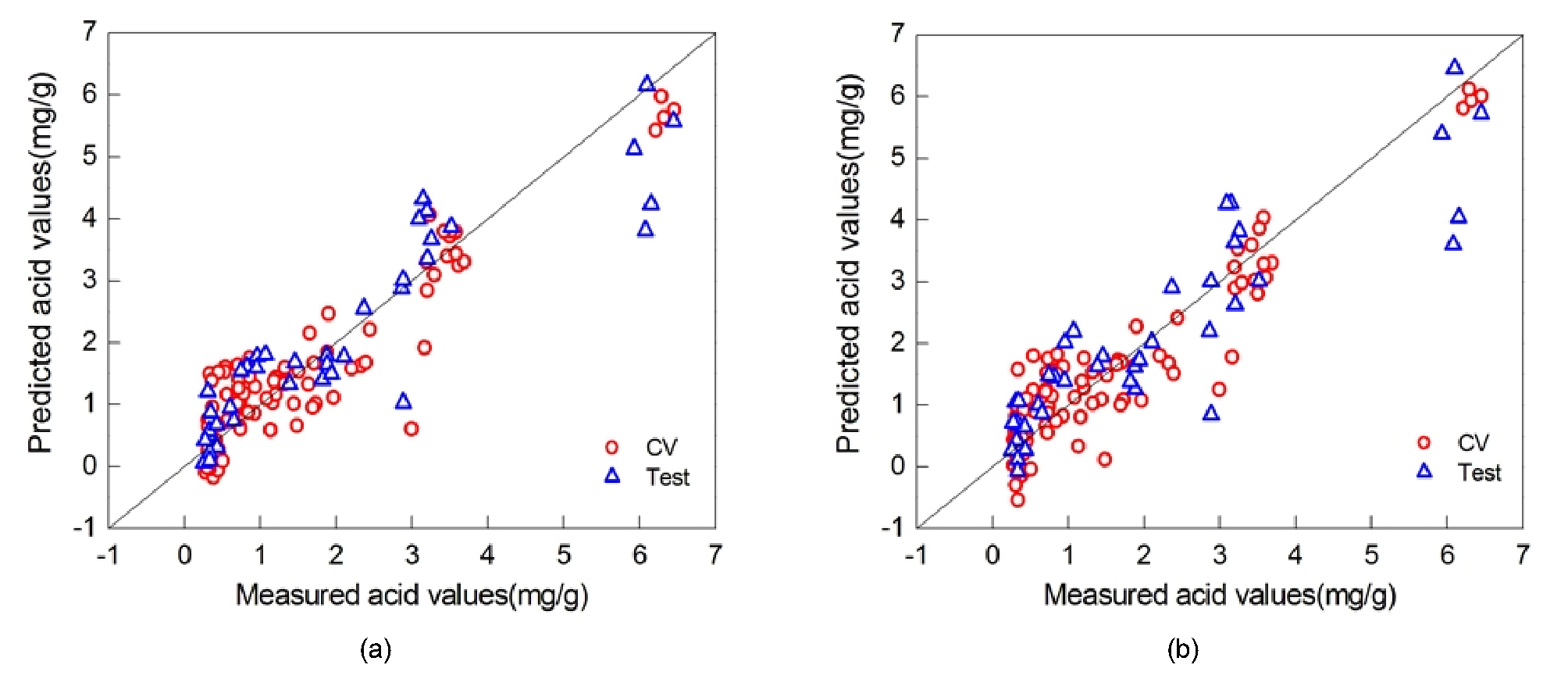##### Figure 7.

PLSR results for (a) bootstrap and (b) VIP optimal variable selection methods.

## Conclusions

In this paper, we demonstrated the feasibility of predicting the rancidity of soybean oil (using its acid value) by means of a near-infrared spectroscopy technique and optimal variable selection methods. While developing the PLSR model, we successfully used suitable preprocessing methods to remove the noise properties associated with transmission spectra. We investigated seven processing methods, and we found that the Savitzky-Golay 2nd order derivative method exhibited the best performance in estimating the acid value. Furthermore, we employed the optimal variable selection methods because the spectra information corresponding to the entire wavelength is likely to reduce the model accuracy. Results showed that both methods of bootstrap and VIP seems to be the good variable selector to extract the optimal spectra but not enhanced in this study.

It is commonly known that NIRS techniques can be used in both qualitative and quantitative analysis of various objects owing to their mechanisms related to the vibrational energy of molecules. Moreover, this NIR method, being non-destructive and remote sensing, has a lot of advantages, especially in simplifying the activities at the work spot; however, it has a specific limitation in respect of the noise from the instrument and its environment. In order to overcome these drawbacks, multivariate analysis such as PLSR technique with optimal variable selection methods and proper preprocessing methods have been studied and developed similar to our study. We expect that the results of this NIRS study about modeling and statistical evaluations can potentially provide good opportunities for multivariable analysis of agricultural products and foods, as well as many other scientific area.

## Conflict of Interest

The authors have no conflicting financial or other interests.

## Acknowledgements

This study was carried out with the support of “Cooperative Research Program for Agricultural Sciences & Technology Development (Project No. PJ012759032018)”, Rural Development Administration, Republic of Korea.

## References

1

AOCS. 1977. Official Methods and Recommended Practices of the American Oil Chemists’ Society, method Te 1a-64. Champaign, IL, USA: American Oil Chemists' Society.

2

ASTM International. 2000. American Society for Testing and Materials, E1866-97: Standard Guide for Establishing Spectrophotometer Performance Tests; E1655: Standard Practices for Infrared, Multivariate, Quantitative Analysis. Philadelphia, PA, USA: Official ASTM Publications.

3

Ben-Dor, E., J. R. Irons and G. F. Epema. 1999. Remote Sensing for the Earth Sciences: Manual of Remote Sensing. 3rd ed. New York: John Wiley & Sons.

4

Bickel, P. J. and D. A. Freedman. 1981. Some Asymptotic Theory for the Bootstrap. The Annals of Statistics 9(6): 1196-1217.https://projecteuclid.org/euclid.aos/1176345637

10.1214/aos/1176345637
5

Choe, E. and D. B. Min. 2006. Mechanisms and factors for edible oil oxidation. Comprehensive reviews in food science and food safety 5(4): 169-186. https://doi.org/10.1111/j.1541-4337.2006.00009.x

10.1111/j.1541-4337.2006.00009.x
6

Choe, E., F. van der Meer, F. van Ruitenbeek, H. van der Werff, B. de Smeth, and K. W. Kim. 2008. Mapping of heavy metal pollution in stream sediments using combined geochemistry, field spectroscopy, and hyperspectral remote sensing: a case study of the Rodalquilar mining area, SE Spain. Remote Sensing of Environment 112(7): 3222-3233.https://doi.org/10.1016/j.rse.2008.03.017

10.1016/j.rse.2008.03.017
7

Chung, H.I. and H.J. Kim. 2000. Near-Infrared spectroscopy: principles. Analytical science & technology 13(1): 138-151. (In Korean, with English abstract).

8

Chung, H. K., C. S. Choe, J. H. Lee, M. J. Chang, and M. H. Kang. 2003. Oxidative stability of the pine needle extracted oils and sensory evaluation of savored laver made by extracted oils. Journal of the Korean Society of Food Culture 18(2): 89-95.

9

Dull, G.G., R.G. Leffler, G.S. Birth and D.A. Smittle. 1992. Instrument for non-destructive measurement of soluble solids in honeydew melons. Transactions of American Society of Agricultural Engineers 35: 735-737.http://elibrary.asabe.org/azdez.asp?AID=28656&T=2

10

Geladi, P. and B. R. Kowalski. 1986. Partial least-squares regression: a tutorial. Analytica chimica acta 185: 1-17.https://doi.org/10.1016/0003-2670(86)80028-9

10.1016/0003-2670(86)80028-9
11

Helland, I. S. 1988. On the structure of partial least squares regression. Communications in statistics- Simulation and Computation 17(2): 581-607.https://doi.org/10.1080/03610918808812681

10.1080/03610918808812681
12

Joshi, R., C. Mo, W.-H. Lee, S. H. Lee and B.-K. Cho. 2015. Review of rice quality under various growth and storage conditions and its evaluation using spectroscopic technology. Journal of Biosystems Engineering 40(2): 124-136.https://doi.org/10.5307/JBE.2015.40.2.124

10.5307/JBE.2015.40.2.124
13

Kamruzzaman, M., G. ElMasry, D.-W. Sun and P. Allen. 2013. Non-destructive assessment of instrumental and sensory tenderness of lamb meat using NIR hyperspectral imaging. Food Chemistry 141(1): 389-396.

10.1016/j.foodchem.2013.02.09423768372
14

Kandpal, L.M., S. Lohumi, M.S. Kim, J.-S. Kang and B.-K, Cho. 2016. Near-Infrared hyperspectral imaging system coupled with multivariate methods to predict viability and vigor in muskmelon seeds. Sensors and Actuators B: Chemical 229: 534-544.https://doi.org/10.1016/j.snb.2016.02.015

10.1016/j.snb.2016.02.015
15

Kawano, S., H. Watanabe and M. Iwamoto. 1992. Determination of sugar content in intact peaches by near infrared spectroscopy journal of Japanese society of horticultural science 61(2): 445-451.https://doi.org/10.2503/jjshs.61.445

10.2503/jjshs.61.445
16

Kim, Y.T. and S.R. Suh. 2008. Comparison of performance of models to predict hardness of tomato using spectroscopic data of reflectance and transmittance. Journal of Biosystems Engineering 33(1): 63-68. (In Korean, with English abstract)https://doi.org/10.5307/JBE.2008.33.1.063

10.5307/JBE.2008.33.1.063
17

Kweon, G. Y., E. Lund, C. Maxton, P. Drummond and K. Jensen. 2009. Soil profile measurement of carbon contents using a probe-type VIS-NIR spectrophotometer. Journal of Biosystems Engineering 34(5): 382-389.https://www.e-sciencecentral.org/articles/SC000015422

18

Lazraq, A., R. Cléroux and J.-P. Gauchi. 2003. Selecting both latent and explanatory variables in the PLS1 regression model. Chemometrics and Intelligent Laboratory Systems 66(2): 117-126.https://doi.org/10.1016/S0169-7439(03)00027-3

10.1016/S0169-7439(03)00027-3
19

Lee, C.J., H.W. Park, C.O. Huh, G.Y. Kim, M.H. Kang, S.S. Cho and S.J. Kim. 1997. A study on the using frequency of edible oils and food development by using edible oil in food service operation. Food Industry and Nutrition. 2: 33-40. (In Korean, with English abstract).

20

Lee, K.J., W. R. Hruschka, J. A. Abbott, S. H. Noh and B. S. Park. 1998, Predicting the soluble solids of apples by near infrared spectroscopy (Ⅱ) –PLS and ANN Models-. Journal of Biosystems Engineering 23(6): 571-582. (In Korean, with English abstract).http://www.koreascience.or.kr/article/ArticleFullRecord.jsp?cn=NOGGB5_1998_v23n6_571

21

May, W. A., R. J. Peterson, and S. S. Chang. 1983. Chemical reactions involved in the deep-fat frying of foods: IX. Identification of the volatile decomposition products of triolein. Journal of the American Oil Chemists’ Society 60(5): 990-995.https://link.springer.com/article/10.1007/BF02660214

22

Mehmood, T., K. H. Liland, L. Snipen and S. Sæbø. 2012. A review of variable selection methods in partial least squares regression. Chemometrics and Intelligent Laboratory Systems 118: 62-69.https://doi.org/10.1016/j.chemolab.2012.07.010

10.1016/j.chemolab.2012.07.010
23

Park, G.Y., A.K. Kim, K.A. Park, B.K. Jung, C.H. Bae and M.H. Kim. 2003. Acidification of frying oil used for chicken. Journal of Food Hygiene and Safety 18(1): 36-41. (In Korean, with English abstract).http://db.koreascholar.com/article.aspx?code=344259

24

Porep, J. U., D. R. Kammerer and R, Carle. 2015. On-line application of near infrared (NIR) spectroscopy in food production. Trends in Food Science & Technology 46(2): 211-230.https://doi.org/10.1016/j.tifs.2015.10.002

10.1016/j.tifs.2015.10.002
25

Rinnan, A., F. van den Berg and S.B. Engelsen. 2009. Review of the most common pre-processing techniques for near-infrared spectra. Trends in Analytical Chemistry 28(10): 1201-1222.https://doi.org/10.1016/j.trac.2009.07.007

10.1016/j.trac.2009.07.007
26

Sarathjith, M.C., B.S. Das, S. P. Wani and K.L. Sahrawat. 2016. Variable indicators for optimum wavelength selection in diffuse reflectance spectroscopy of soils. Geoderma 267(1): 1-9.https://doi.org/10.1016/j.geoderma.2015.12.031

10.1016/j.geoderma.2015.12.031
27

Summerfield, F. W and A. L. Tappel, “Detection and measurement by high-performance liquid chromatography of malondialdehyde crosslinks in DNA,” Analytical Biochemistry, vol. 143, no. 2, pp. 265-271, 1984.https://doi.org/10.1016/0003-2697(84)90662-6

10.1016/0003-2697(84)90662-6
28

Wold, S., M. Sjöström and L. Eriksson. 2001. PLS- regression: a basic tool of chemometrics. Chemometrics and intelligent laboratory systems 58(2): 109-130.https://doi.org/10.1016/S0169-7439(01)00155-1

10.1016/S0169-7439(01)00155-1
29

Zhang, C., H. Jiang, F. Liu and Y. He. 2017. Application of near-infrared hyperspectral imaging with variable selection methods to determine and visualize caffeine content of coffee beans. Food and Bioprocess Technology 10(1): 213-221.https://link.springer.com/article/10.1007/s11947-016-1809-8